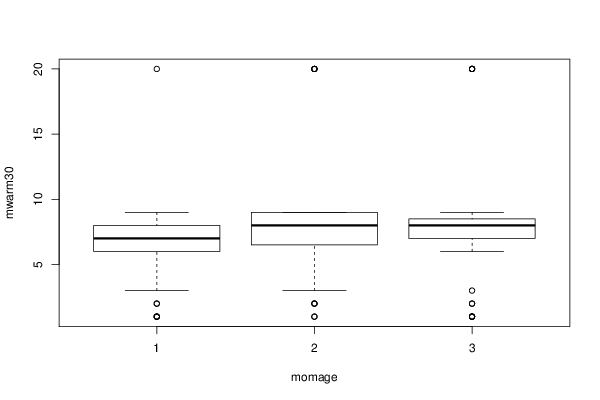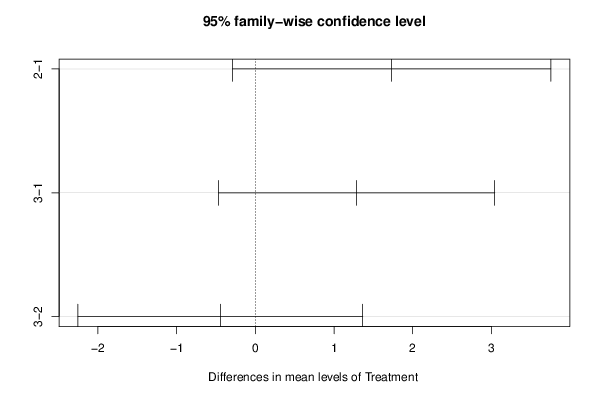## Free Statistics

of Irreproducible Research!

Author's title
Author*The author of this computation has been verified*
R Software Modulerwasp_One Factor ANOVA.wasp
Title produced by softwareOne-Way-Between-Groups ANOVA- Free Statistics Software (Calculator)
Date of computationMon, 05 Nov 2012 19:41:41 -0500
Cite this page as followsStatistical Computations at FreeStatistics.org, Office for Research Development and Education, URL https://freestatistics.org/blog/index.php?v=date/2012/Nov/05/t1352162624l1c6sphpb8ibkh8.htm/, Retrieved Mon, 25 Sep 2023 22:44:12 +0000
Statistical Computations at FreeStatistics.org, Office for Research Development and Education, URL https://freestatistics.org/blog/index.php?pk=186419, Retrieved Mon, 25 Sep 2023 22:44:12 +0000
QR Codes:Original text written by user:
IsPrivate?No (this computation is public)
User-defined keywords
Estimated Impact113
Family? (F = Feedback message, R = changed R code, M = changed R Module, P = changed Parameters, D = changed Data)
-     [Chi Square Measure of Association- Free Statistics Software (Calculator)] [One Way ANOVA wit...] [2009-11-29 13:09:19] [98fd0e87c3eb04e0cc2efde01dbafab6]
-   PD  [Chi Square Measure of Association- Free Statistics Software (Calculator)] [One Way ANOVA for...] [2009-12-01 13:05:10] [3fdd735c61ad38cbc9b3393dc997cdb7]
- R P     [Chi Square Measure of Association- Free Statistics Software (Calculator)] [CARE date with Tu...] [2009-12-01 18:33:48] [98fd0e87c3eb04e0cc2efde01dbafab6]
-   P       [One-Way-Between-Groups ANOVA- Free Statistics Software (Calculator)] [CARE Data with Tu...] [2010-11-23 12:09:38] [3fdd735c61ad38cbc9b3393dc997cdb7]
- RM          [One-Way-Between-Groups ANOVA- Free Statistics Software (Calculator)] [IQ and Mothers Age] [2011-11-21 16:34:08] [98fd0e87c3eb04e0cc2efde01dbafab6]
- R  D            [One-Way-Between-Groups ANOVA- Free Statistics Software (Calculator)] [workshop 5: mwarm...] [2012-11-06 00:41:41] [b9bb0bee71e79baedbda8d04cb7f9b59] [Current]
-    D              [One-Way-Between-Groups ANOVA- Free Statistics Software (Calculator)] [workshop 5: mc30v...] [2012-11-06 00:53:59] [371dda6194462372473caa1f809126cb]
-    D              [One-Way-Between-Groups ANOVA- Free Statistics Software (Calculator)] [workshop 5: wiscr...] [2012-11-06 00:58:15] [371dda6194462372473caa1f809126cb]
-   PD                [One-Way-Between-Groups ANOVA- Free Statistics Software (Calculator)] [workshop 5: mvrbi...] [2012-11-06 01:16:04] [371dda6194462372473caa1f809126cb]
-   PD                  [One-Way-Between-Groups ANOVA- Free Statistics Software (Calculator)] [workshop 5: MWARM...] [2012-11-06 01:31:54] [371dda6194462372473caa1f809126cb]
Feedback Forum

Post a new message
Dataseries X:
3	3
6	2
8	3
8	3
7	3
1	2
7	1
8	3
9	3
9	1
3	2
9	2
7	3
9	3
8	3
6	2
7	3
8	3
9	3
7	1
6	1
8	2
7	2
7	3
8	3
9	2
9	2
7	3
2	2
7	1
7	3
9	1
7	1
9	3
20	2
1	3
6	3
9	2
9	1
8	2
6	2
6	1
1	2
8	2
8	3
1	1
6	1
9	2
8	2
2	2
8	2
9	1
7	3
7	3
6	3
9	3
9	1
8	1
2	1
6	3
20	3
8	1
7	1
7	1
8	3
3	1
8	3
20	3
7	1
1	1
20	3
1	3
8	1
9	2
6	1
9	1
8	1
1	1
8	3
3	1
7	3
8	2
20	3
9	3
20	2
9	2
8	1
8	2
8	3
9	2
2	3
6	1
7	3
2	2
9	2
7	1
8	3
8	2
8	3
7	2
7	1
9	1
8	3
8	3
9	2
9	3
20	3
7	3
8	1
1	3
9	3
8	3
7	3
8	2
8	3
7	2
6	3
7	3
7	3
6	1
6	3
7	1
9	3
6	1
20	1
2	1
8	3
7	3
20	2
6	2
1	3
9	3
8	1
9	2
8	3
8	3
9	3
8	1
9	2
7	3
6	3
8	3
6	1
1	3
3	1
6	3
8	3
7	1
8	3
6	3
9	3
9	3
20	2
7	3
1	1
8	3
9	3
8	2
8	2
2	3

 Summary of computational transaction Raw Input view raw input (R code) Raw Output view raw output of R engine Computing time 2 seconds R Server 'Gertrude Mary Cox' @ cox.wessa.net

\begin{tabular}{lllllllll}
\hline
Summary of computational transaction \tabularnewline
Raw Input & view raw input (R code)  \tabularnewline
Raw Output & view raw output of R engine  \tabularnewline
Computing time & 2 seconds \tabularnewline
R Server & 'Gertrude Mary Cox' @ cox.wessa.net \tabularnewline
\hline
\end{tabular}
%Source: https://freestatistics.org/blog/index.php?pk=186419&T=0

[TABLE]
[ROW][C]Summary of computational transaction[/C][/ROW]
[ROW][C]Raw Input[/C][C]view raw input (R code) [/C][/ROW]
[ROW][C]Raw Output[/C][C]view raw output of R engine [/C][/ROW]
[ROW][C]Computing time[/C][C]2 seconds[/C][/ROW]
[ROW][C]R Server[/C][C]'Gertrude Mary Cox' @ cox.wessa.net[/C][/ROW]
[/TABLE]
Source: https://freestatistics.org/blog/index.php?pk=186419&T=0

Globally Unique Identifier (entire table): ba.freestatistics.org/blog/index.php?pk=186419&T=0

As an alternative you can also use a QR Code:

The GUIDs for individual cells are displayed in the table below:

 Summary of computational transaction Raw Input view raw input (R code) Raw Output view raw output of R engine Computing time 2 seconds R Server 'Gertrude Mary Cox' @ cox.wessa.net

 ANOVA Model mwarm30 ~ momage means 6.568 1.732 1.287

\begin{tabular}{lllllllll}
\hline
ANOVA Model \tabularnewline
mwarm30  ~  momage \tabularnewline
means & 6.568 & 1.732 & 1.287 \tabularnewline
\hline
\end{tabular}
%Source: https://freestatistics.org/blog/index.php?pk=186419&T=1

[TABLE]
[ROW][C]ANOVA Model[/C][/ROW]
[ROW][C]mwarm30  ~  momage[/C][/ROW]
[ROW][C]means[/C][C]6.568[/C][C]1.732[/C][C]1.287[/C][/ROW]
[/TABLE]
Source: https://freestatistics.org/blog/index.php?pk=186419&T=1

Globally Unique Identifier (entire table): ba.freestatistics.org/blog/index.php?pk=186419&T=1

As an alternative you can also use a QR Code:

The GUIDs for individual cells are displayed in the table below:

 ANOVA Model mwarm30 ~ momage means 6.568 1.732 1.287

 ANOVA Statistics Df Sum Sq Mean Sq F value Pr(>F) momage 2 71.372 35.686 2.33 0.101 Residuals 157 2404.603 15.316

\begin{tabular}{lllllllll}
\hline
ANOVA Statistics \tabularnewline
& Df & Sum Sq & Mean Sq & F value & Pr(>F) \tabularnewline
momage & 2 & 71.372 & 35.686 & 2.33 & 0.101 \tabularnewline
Residuals & 157 & 2404.603 & 15.316 &   &   \tabularnewline
\hline
\end{tabular}
%Source: https://freestatistics.org/blog/index.php?pk=186419&T=2

[TABLE]
[ROW][C]ANOVA Statistics[/C][/ROW]
[ROW][C] [/C][C]Df[/C][C]Sum Sq[/C][C]Mean Sq[/C][C]F value[/C][C]Pr(>F)[/C][/ROW]
[ROW][C]momage[/C][C]2[/C][C]71.372[/C][C]35.686[/C][C]2.33[/C][C]0.101[/C][/ROW]
[ROW][C]Residuals[/C][C]157[/C][C]2404.603[/C][C]15.316[/C][C] [/C][C] [/C][/ROW]
[/TABLE]
Source: https://freestatistics.org/blog/index.php?pk=186419&T=2

Globally Unique Identifier (entire table): ba.freestatistics.org/blog/index.php?pk=186419&T=2

As an alternative you can also use a QR Code:

The GUIDs for individual cells are displayed in the table below:

 ANOVA Statistics Df Sum Sq Mean Sq F value Pr(>F) momage 2 71.372 35.686 2.33 0.101 Residuals 157 2404.603 15.316

 Tukey Honest Significant Difference Comparisons diff lwr upr p adj 2-1 1.732 -0.291 3.755 0.109 3-1 1.287 -0.467 3.041 0.195 3-2 -0.445 -2.254 1.364 0.83

\begin{tabular}{lllllllll}
\hline
Tukey Honest Significant Difference Comparisons \tabularnewline
& diff & lwr & upr & p adj \tabularnewline
2-1 & 1.732 & -0.291 & 3.755 & 0.109 \tabularnewline
3-1 & 1.287 & -0.467 & 3.041 & 0.195 \tabularnewline
3-2 & -0.445 & -2.254 & 1.364 & 0.83 \tabularnewline
\hline
\end{tabular}
%Source: https://freestatistics.org/blog/index.php?pk=186419&T=3

[TABLE]
[ROW][C]Tukey Honest Significant Difference Comparisons[/C][/ROW]
[ROW][C]2-1[/C][C]1.732[/C][C]-0.291[/C][C]3.755[/C][C]0.109[/C][/ROW]
[ROW][C]3-1[/C][C]1.287[/C][C]-0.467[/C][C]3.041[/C][C]0.195[/C][/ROW]
[ROW][C]3-2[/C][C]-0.445[/C][C]-2.254[/C][C]1.364[/C][C]0.83[/C][/ROW]
[/TABLE]
Source: https://freestatistics.org/blog/index.php?pk=186419&T=3

Globally Unique Identifier (entire table): ba.freestatistics.org/blog/index.php?pk=186419&T=3

As an alternative you can also use a QR Code:

The GUIDs for individual cells are displayed in the table below:

 Tukey Honest Significant Difference Comparisons diff lwr upr p adj 2-1 1.732 -0.291 3.755 0.109 3-1 1.287 -0.467 3.041 0.195 3-2 -0.445 -2.254 1.364 0.83

 Levenes Test for Homogeneity of Variance Df F value Pr(>F) Group 2 0.592 0.555 157

\begin{tabular}{lllllllll}
\hline
Levenes Test for Homogeneity of Variance \tabularnewline
& Df & F value & Pr(>F) \tabularnewline
Group & 2 & 0.592 & 0.555 \tabularnewline
& 157 &   &   \tabularnewline
\hline
\end{tabular}
%Source: https://freestatistics.org/blog/index.php?pk=186419&T=4

[TABLE]
[ROW][C]Levenes Test for Homogeneity of Variance[/C][/ROW]
[ROW][C] [/C][C]Df[/C][C]F value[/C][C]Pr(>F)[/C][/ROW]
[ROW][C]Group[/C][C]2[/C][C]0.592[/C][C]0.555[/C][/ROW]
[ROW][C] [/C][C]157[/C][C] [/C][C] [/C][/ROW]
[/TABLE]
Source: https://freestatistics.org/blog/index.php?pk=186419&T=4

Globally Unique Identifier (entire table): ba.freestatistics.org/blog/index.php?pk=186419&T=4

As an alternative you can also use a QR Code:

The GUIDs for individual cells are displayed in the table below:

 Levenes Test for Homogeneity of Variance Df F value Pr(>F) Group 2 0.592 0.555 157PNG link Postscript link PDF linkPNG link Postscript link PDF link

Parameters (Session):
par1 = 1 ; par2 = 2 ; par3 = TRUE ;
Parameters (R input):
par1 = 1 ; par2 = 2 ; par3 = TRUE ;
R code (references can be found in the software module):
cat1 <- as.numeric(par1) #cat2<- as.numeric(par2) #intercept<-as.logical(par3)x <- t(x)x1<-as.numeric(x[,cat1])f1<-as.character(x[,cat2])xdf<-data.frame(x1,f1)(V1<-dimnames(y)[][cat1])(V2<-dimnames(y)[][cat2])names(xdf)<-c('Response', 'Treatment')if(intercept == FALSE) (lmxdf<-lm(Response ~ Treatment - 1, data = xdf) ) else (lmxdf<-lm(Response ~ Treatment, data = xdf) )(aov.xdf<-aov(lmxdf) )(anova.xdf<-anova(lmxdf) )load(file='createtable')a<-table.start()a<-table.row.start(a)a<-table.element(a,'ANOVA Model', length(lmxdf$coefficients)+1,TRUE)a<-table.row.end(a)a<-table.row.start(a)a<-table.element(a, paste(V1, ' ~ ', V2), length(lmxdf$coefficients)+1,TRUE)a<-table.row.end(a)a<-table.row.start(a)a<-table.element(a, 'means',,TRUE)for(i in 1:length(lmxdf$coefficients)){a<-table.element(a, round(lmxdf$coefficients[i], digits=3),,FALSE)}a<-table.row.end(a)a<-table.end(a)table.save(a,file='mytable.tab')a<-table.start()a<-table.row.start(a)a<-table.element(a,'ANOVA Statistics', 5+1,TRUE)a<-table.row.end(a)a<-table.row.start(a)a<-table.element(a, ' ',,TRUE)a<-table.element(a, 'Df',,FALSE)a<-table.element(a, 'Sum Sq',,FALSE)a<-table.element(a, 'Mean Sq',,FALSE)a<-table.element(a, 'F value',,FALSE)a<-table.element(a, 'Pr(>F)',,FALSE)a<-table.row.end(a)a<-table.row.start(a)a<-table.element(a, V2,,TRUE)a<-table.element(a, anova.xdf$Df,,FALSE)a<-table.element(a, round(anova.xdf$'Sum Sq', digits=3),,FALSE)a<-table.element(a, round(anova.xdf$'Mean Sq', digits=3),,FALSE)a<-table.element(a, round(anova.xdf$'F value', digits=3),,FALSE)a<-table.element(a, round(anova.xdf$'Pr(>F)', digits=3),,FALSE)a<-table.row.end(a)a<-table.row.start(a)a<-table.element(a, 'Residuals',,TRUE)a<-table.element(a, anova.xdf$Df,,FALSE)a<-table.element(a, round(anova.xdf$'Sum Sq', digits=3),,FALSE)a<-table.element(a, round(anova.xdf$'Mean Sq', digits=3),,FALSE)a<-table.element(a, ' ',,FALSE)a<-table.element(a, ' ',,FALSE)a<-table.row.end(a)a<-table.end(a)table.save(a,file='mytable1.tab')bitmap(file='anovaplot.png')boxplot(Response ~ Treatment, data=xdf, xlab=V2, ylab=V1)dev.off()if(intercept==TRUE){thsd<-TukeyHSD(aov.xdf)bitmap(file='TukeyHSDPlot.png')plot(thsd)dev.off()}if(intercept==TRUE){a<-table.start()a<-table.row.start(a)a<-table.element(a,'Tukey Honest Significant Difference Comparisons', 5,TRUE)a<-table.row.end(a)a<-table.row.start(a)a<-table.element(a, ' ', 1, TRUE)for(i in 1:4){a<-table.element(a,colnames(thsd[])[i], 1, TRUE)}a<-table.row.end(a)for(i in 1:length(rownames(thsd[]))){a<-table.row.start(a)a<-table.element(a,rownames(thsd[])[i], 1, TRUE)for(j in 1:4){a<-table.element(a,round(thsd[][i,j], digits=3), 1, FALSE)}a<-table.row.end(a)}a<-table.end(a)table.save(a,file='mytable2.tab')}if(intercept==FALSE){a<-table.start()a<-table.row.start(a)a<-table.element(a,'TukeyHSD Message', 1,TRUE)a<-table.row.end(a)a<-table.start()a<-table.row.start(a)a<-table.element(a,'Must Include Intercept to use Tukey Test ', 1, FALSE)a<-table.row.end(a)a<-table.end(a)table.save(a,file='mytable2.tab')}library(car)lt.lmxdf<-levene.test(lmxdf)a<-table.start()a<-table.row.start(a)a<-table.element(a,'Levenes Test for Homogeneity of Variance', 4,TRUE)a<-table.row.end(a)a<-table.row.start(a)a<-table.element(a,' ', 1, TRUE)for (i in 1:3){a<-table.element(a,names(lt.lmxdf)[i], 1, FALSE)}a<-table.row.end(a)a<-table.row.start(a)a<-table.element(a,'Group', 1, TRUE)for (i in 1:3){a<-table.element(a,round(lt.lmxdf[[i]], digits=3), 1, FALSE)}a<-table.row.end(a)a<-table.row.start(a)a<-table.element(a,' ', 1, TRUE)a<-table.element(a,lt.lmxdf[], 1, FALSE)a<-table.element(a,' ', 1, FALSE)a<-table.element(a,' ', 1, FALSE)a<-table.row.end(a)a<-table.end(a)table.save(a,file='mytable3.tab')